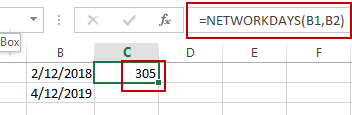# Count the Number of Workdays between Two Dates

This post will guide you how to count the number of workdays between two dates in Excel. How do I get workdays between dates with a formula in Excel. How to calculate the number of working days between two dates in Excel.

## Count the Number of Workdays between Two Dates

If you want to calculate the number of workdays between two dates in Excel, you can use a formula based on the NETWORKDAYS function. And it will exclude all weekend days between the start dates and end dates. You need to specify two dates contain start date and end date so that you can get the number of workdays.

For example, you have two dates in range B1:B2, and you want to get the workdays between these two dates, you just need to use the following formula:

`=NETWORKDAYS(B1,B2)`

Type this formula in a blank Cell, and press Enter key in your keyboard.